﻿ 基于嵌入式技术的电子海图系统硬件设计
 舰船科学技术2022, Vol. 44Issue (10): 119-122    DOI: 10.3404/j.issn.1672-7649.2022.10.024PDF

1. 西安科技大学，陕西 西安 710054;
2. 河南科技职业大学，河南 周口 466000

Hardware design of electronic chart system based on embedded technology
MENG Qing-xun1,2
1. Xi'an University of Science and Technology, Xi′an 710054, China;
2. Henan Vocational University of Science and Technology, Zhoukou 466000, China
Abstract: At present, the embedded electronic chart navigation system has become the mainstream development trend in the field of electronic chart navigation. The system has the characteristics of low cost, powerful functions and stable performance, and is suitable for most ships. In the development of the electronic chart system, the hardware design is the top priority, especially the display module of the electronic chart, which must be paid great attention to. At the same time, in the design of the hardware system, the interference of clutter should be fully considered, so as not to adversely affect the display effect of the chart.
Key words: embedded technology     electronic chart     display module design
0 引　言

1 嵌入式操作系统

2 基于嵌入式技术的电子海图系统硬件设计 2.1 数据结构

MVCF文件中包含了多个子文件，如元数据、图形、索引、属性等，如图1所示。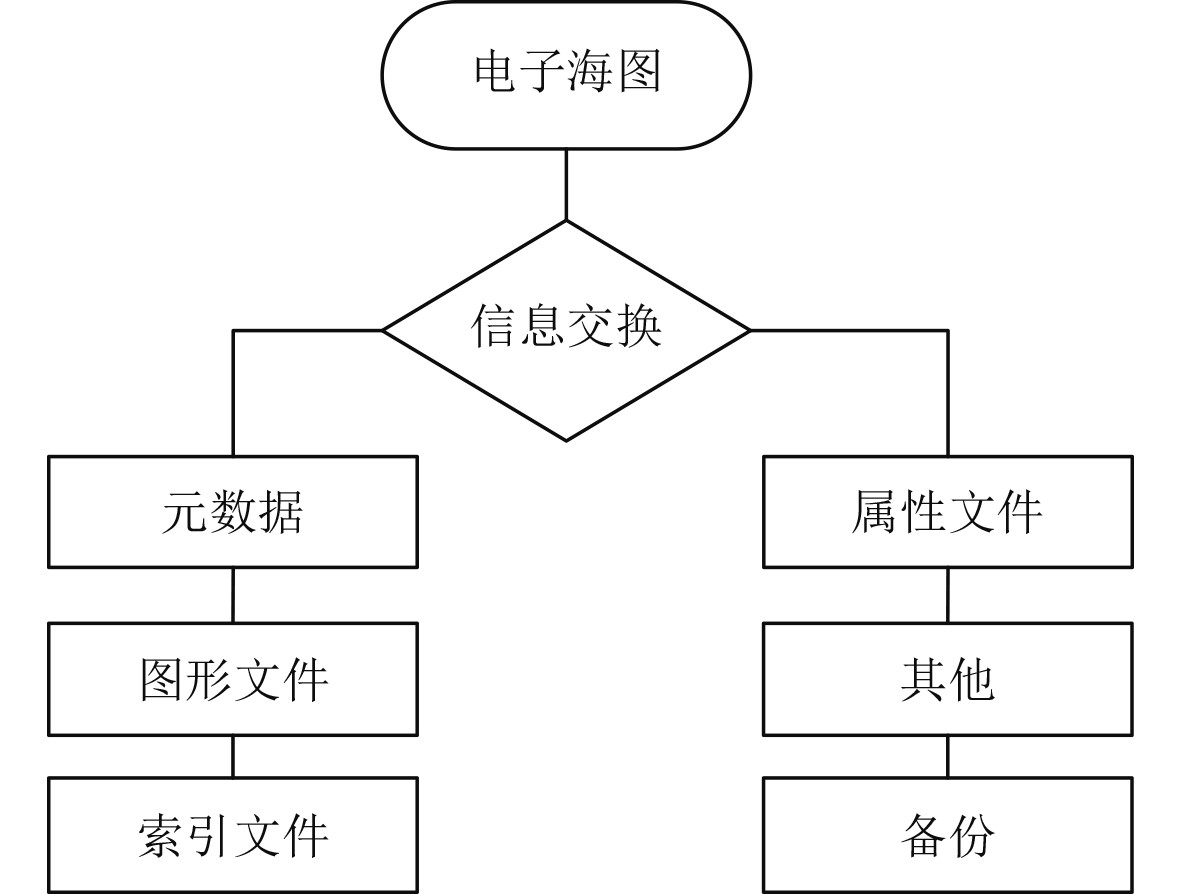图 1 军用矢量格式电子海图组织结构示意图 Fig. 1 Organization structure diagram of military vector format electronic chart

2.2 杂波建模仿真

 $S(f)=\frac{P_{c}}{\sqrt{2 {\text π} \sigma_{f}}} \exp \left[\frac{\left(f-\bar f_{d}\right)^{2}}{2 \sigma_{f}^{2}}\right] \text{。}$

 $R(\tau)=\exp \left(-\alpha \tau^{2}\right) \text{。}$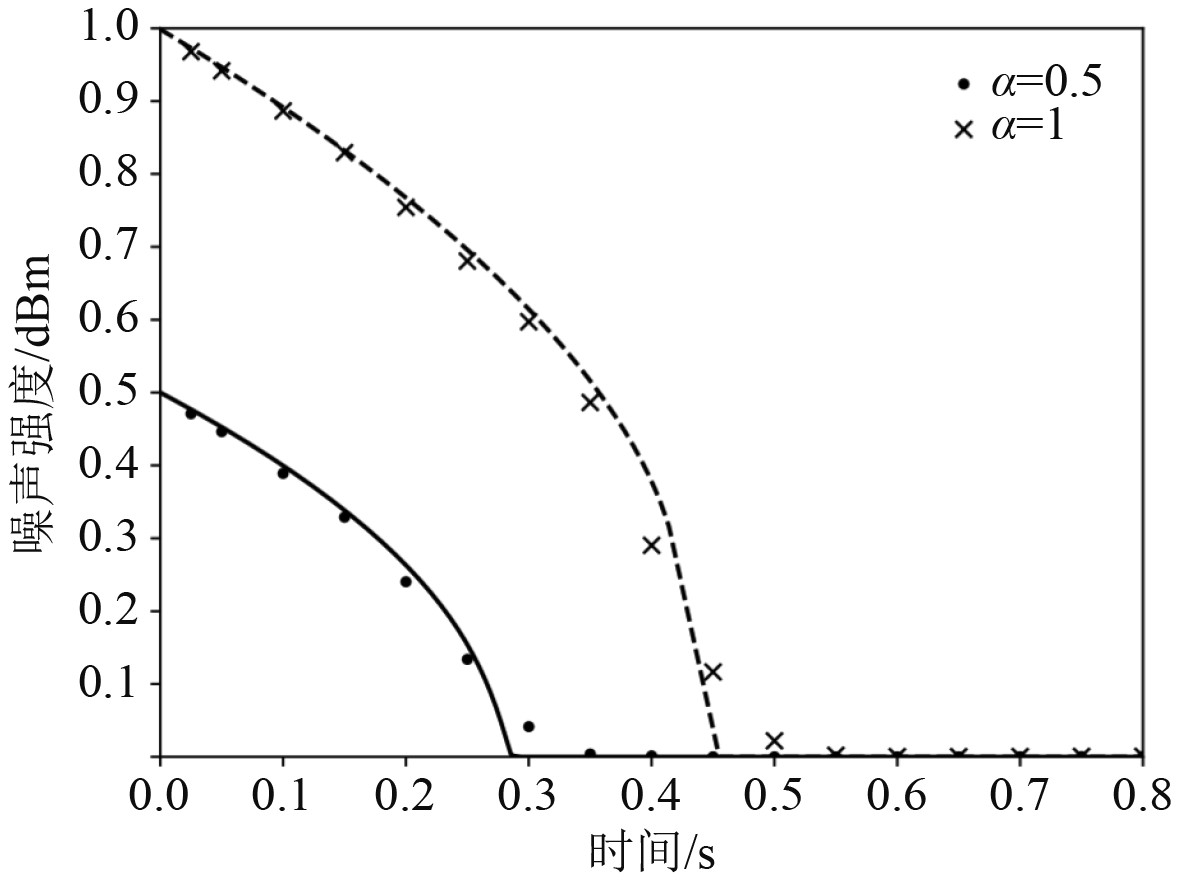图 2 标准高斯分布的噪声信号示意图 Fig. 2 Schematic diagram of noise signal with standard Gaussian distribution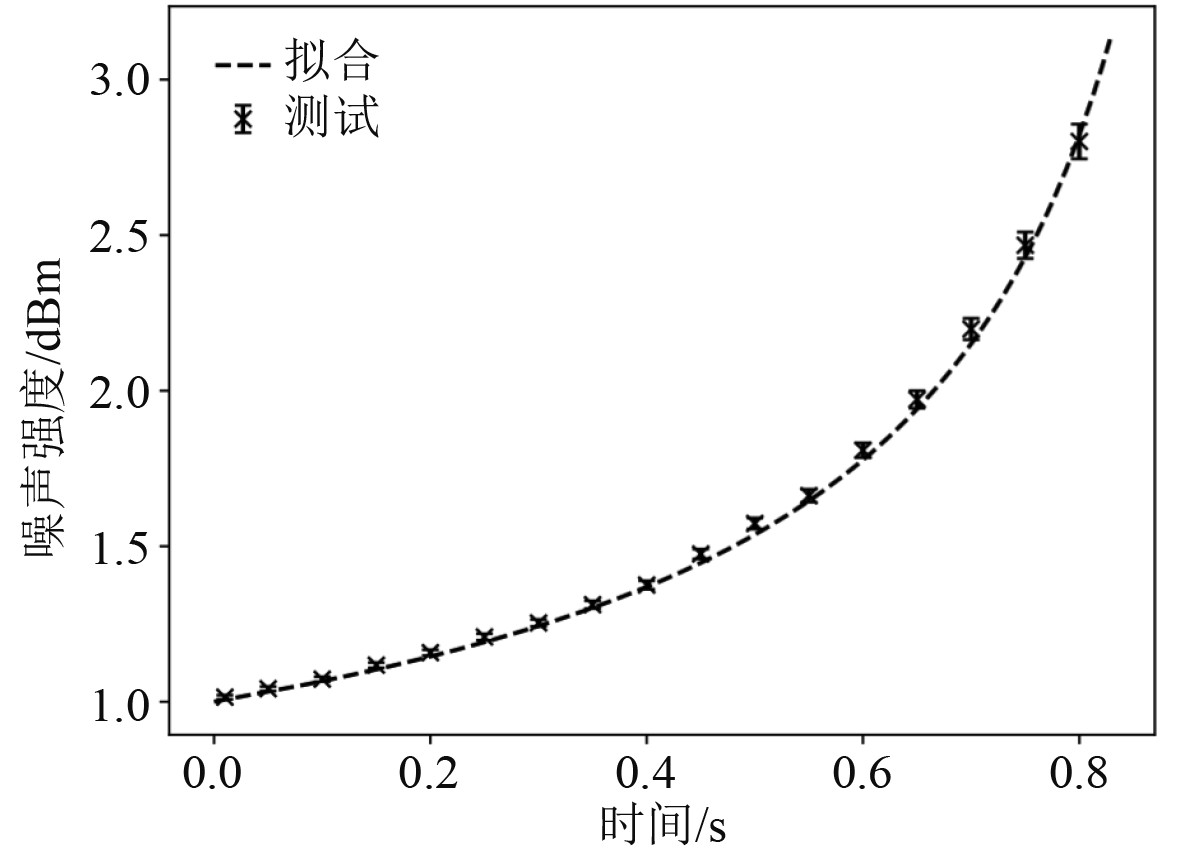图 3 传统高斯分布的噪声强度随时间变化图 Fig. 3 A plot of noise intensity versus time for a traditional Gaussian distribution

 $P_{u u}=P_{v v}=\sigma^{2}\left|H\left(e^{j w}\right)^{2}\right| \text{。}$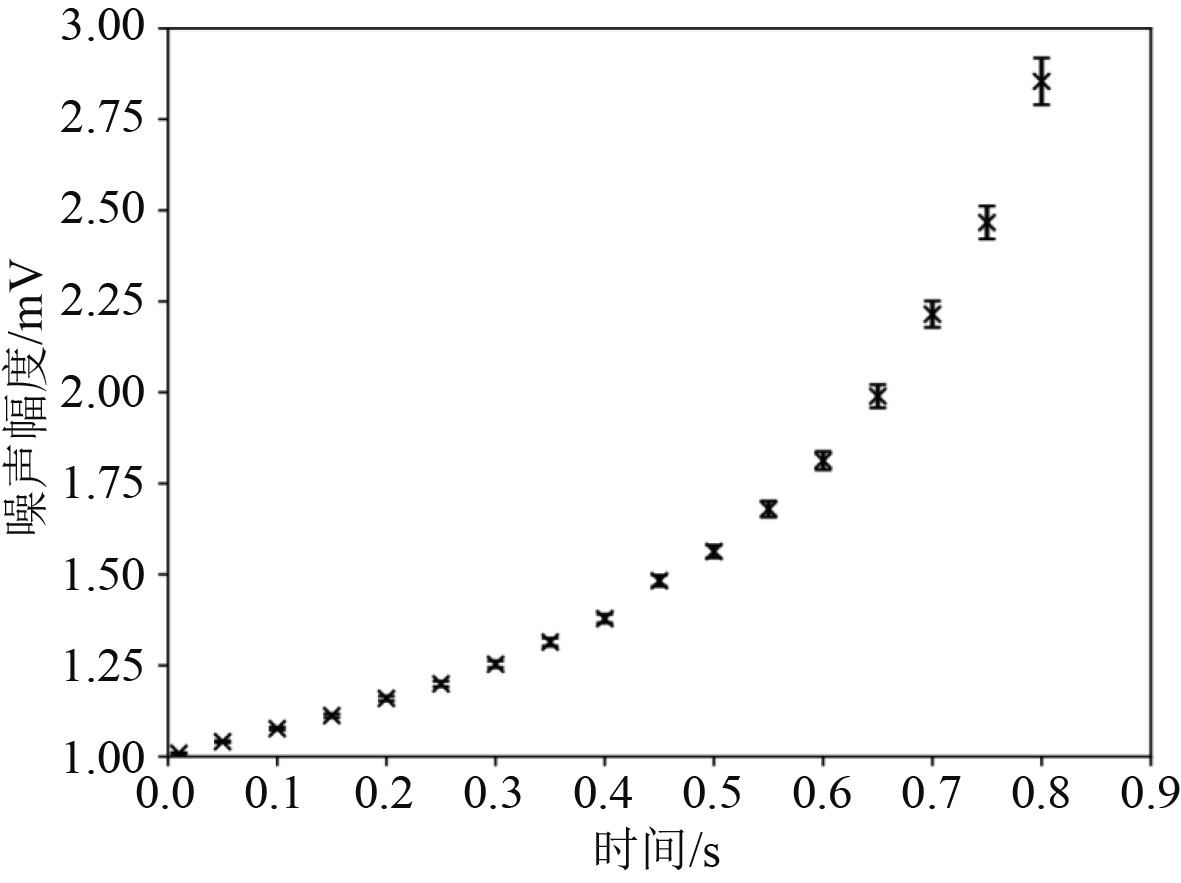图 4 瑞利分布模型中的杂波噪声幅度分布情况示意图 Fig. 4 Schematic diagram of clutter noise amplitude distribution in Rayleigh distribution model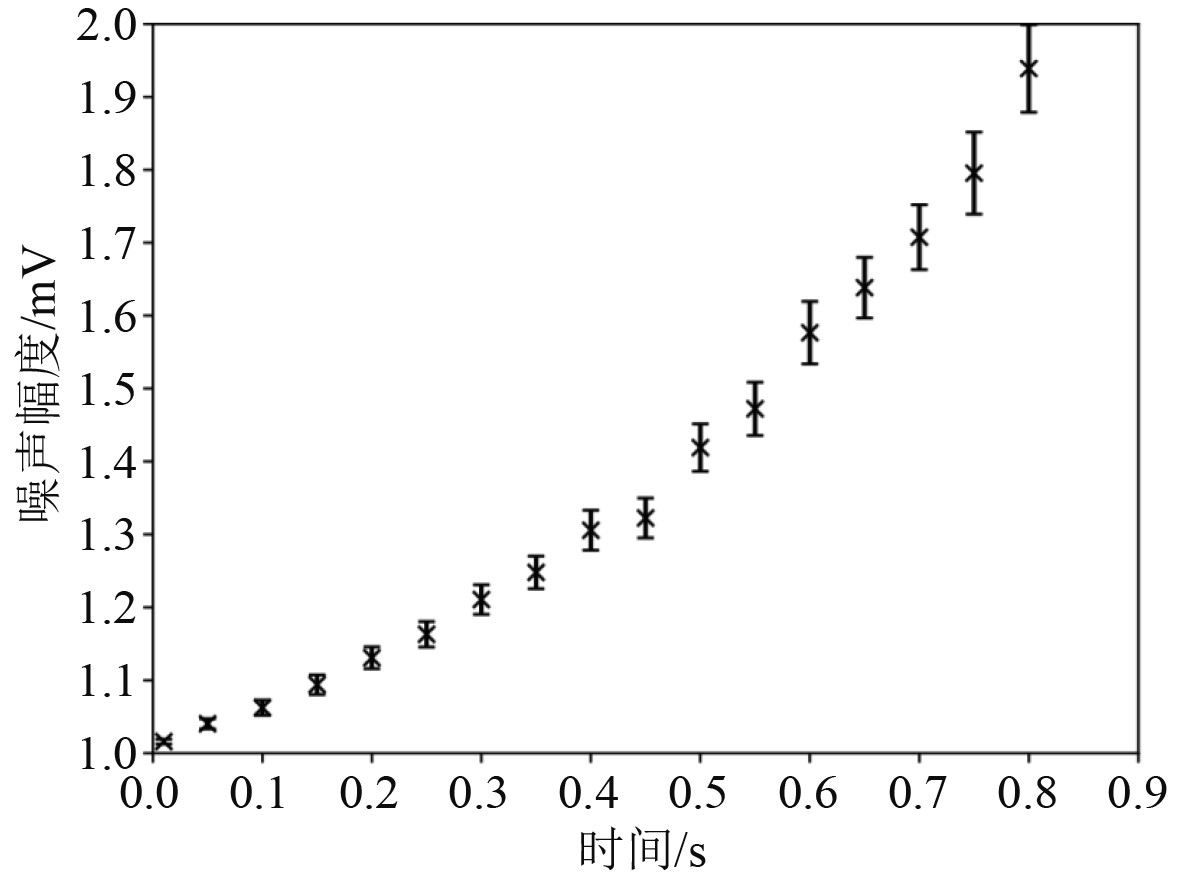图 5 瑞利分布模型中的频谱噪声幅度分布示意图 Fig. 5 Schematic diagram of spectral noise amplitude distribution in Rayleigh distribution model

 ${\kern 1pt} {\kern 1pt} \left\{ x \right\} = {\left[ {u{\kern 1pt} {\kern 1pt} {\kern 1pt} {\kern 1pt} {\kern 1pt} {\kern 1pt} {\kern 1pt} {\kern 1pt} {\kern 1pt} v{\kern 1pt} {\kern 1pt} {\kern 1pt} {\kern 1pt} {\kern 1pt} {\kern 1pt} {\kern 1pt} {\kern 1pt} {\kern 1pt} w} \right]^{\rm{T}}}{\kern 1pt} \text{，}$

 ${\kern 1pt} {\kern 1pt} {\kern 1pt} {\kern 1pt} {\kern 1pt} {\kern 1pt} \left\{ {{\phi _r}} \right\} = {\left[ {\left\{ {\phi _r^u} \right\}{\kern 1pt} {\kern 1pt} {\kern 1pt} {\kern 1pt} {\kern 1pt} {\kern 1pt} {\kern 1pt} {\kern 1pt} {\kern 1pt} \left\{ {\phi _r^v} \right\}{\kern 1pt} {\kern 1pt} {\kern 1pt} {\kern 1pt} {\kern 1pt} {\kern 1pt} {\kern 1pt} {\kern 1pt} {\kern 1pt} \left\{ {\phi _r^w} \right\}} \right]^{\rm{T}}}{\kern 1pt} \text{，}$

 $\left( {\begin{array}{c} \left\{ u \right\} \\ \left\{ v \right\} \\ \left\{ w \right\} \end{array}} \right) = \sum\limits_{r = 1}^m {{q_r}} \left( {\begin{array}{c} \left\{ {\phi _r^u} \right\}\\ \left\{ {\phi _r^v} \right\}\\ \left\{ {\phi _r^w} \right\} \end{array}} \right) \text{。}$

 $\varepsilon = \sum\limits_{r = 1}^m {{q_r}} \psi _r^{{\varepsilon _{}}} \text{。}$

 $\Delta \varepsilon = {\varepsilon ^d} - {\varepsilon ^h} \text{。}$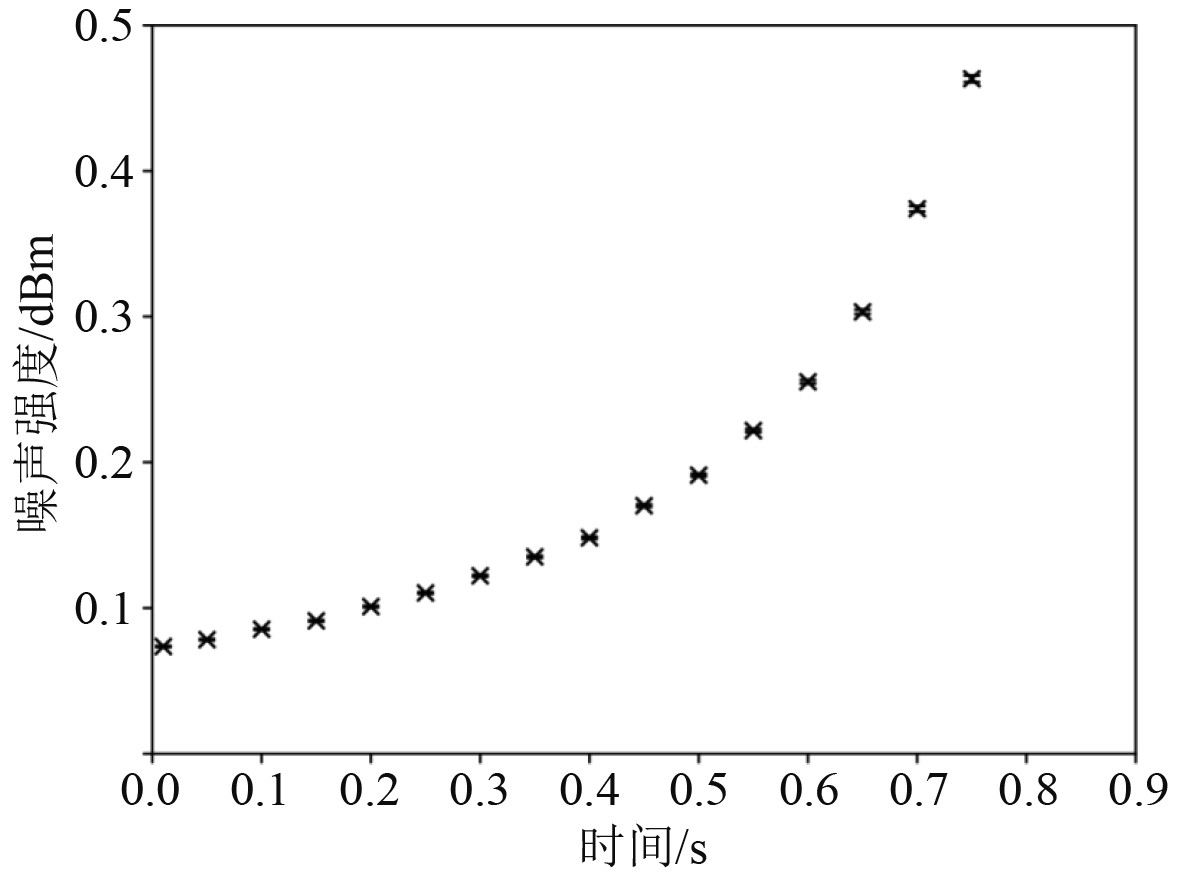图 6 对数正态杂波的时域波形噪声分布情况示意图 Fig. 6 Schematic diagram of the time-domain waveform noise distribution of log-normal clutter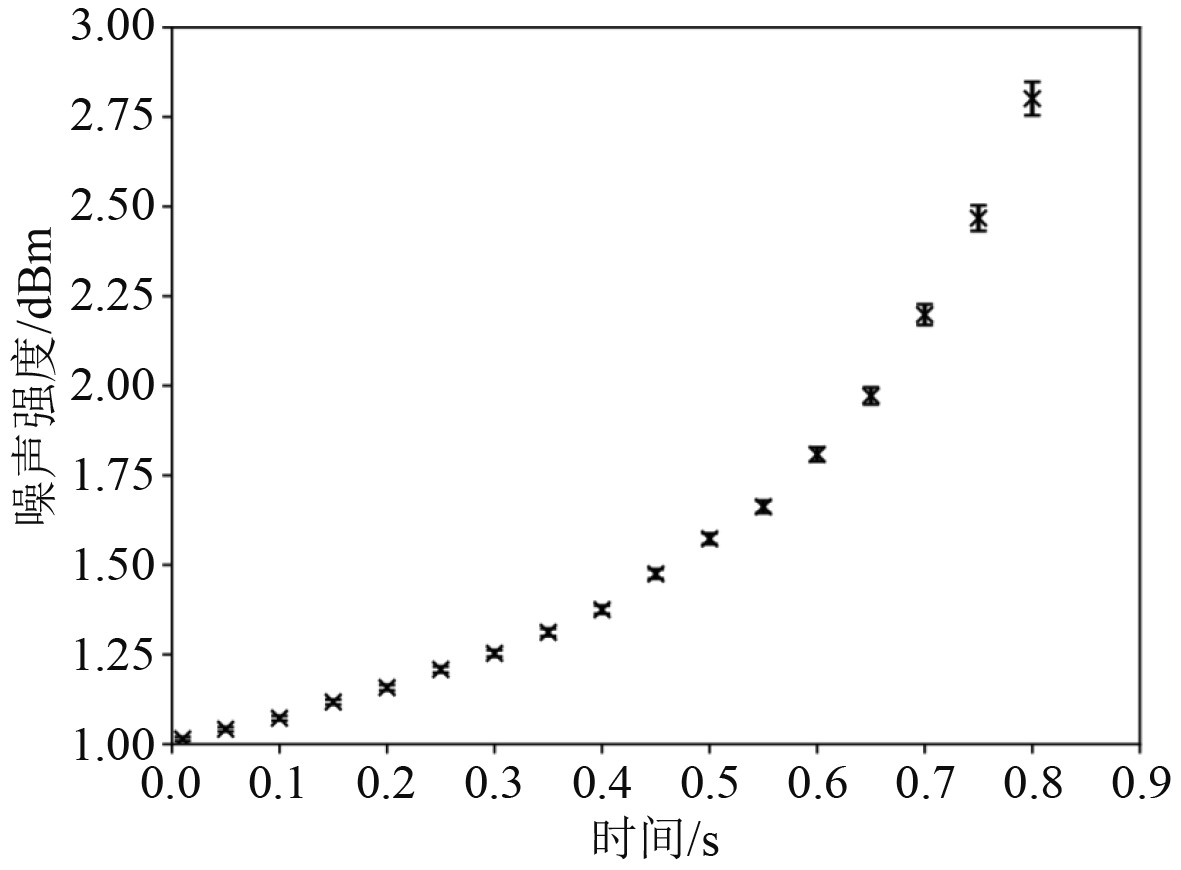图 7 对数正态杂波的频谱噪声情况示意图 Fig. 7 Schematic diagram of spectral noise for log-normal clutter
2.3 设计准备

2.4 显示模块设计

3 结　语

  王玉玺, 丁琰, 肖冲冲. MapInfo数字海图向MVCF数字海图的转换方法研究[J]. 舰船电子工程, 2019, 39(1): 83-84,97. DOI:10.3969/j.issn.1672-9730.2019.01.020  郝江凌, 单雄飞, 赵丽宁, 等. 电子海图导航系统的研究进展与未来趋势[J]. 大连海事大学学报, 2021, 47(3): 1-7.  李荣川, 陈宁. 基于电子海图的船舶路径规划模拟器开发[J]. 机电设备, 2021, 38(4): 9-14.  王百勇, 张艳华, 贾俊乾. 基于深度学习理论下电子海图与雷达图像船舶感知信息融合[J]. 现代雷达, 2021, 43(5): 44-50.  陈明. Douglas-Peucker算法在电子海图岛屿轮廓提取的应用[J]. 电子技术, 2020, 49(5): 36-37.  张大恒, 张英俊, 张闯. 基于Faster R-CNN的电子海图和雷达图像的数据融合[J]. 系统工程与电子技术, 2020, 42(6): 1267-1273.  叶美芬, 陈育才. 基于OGR库的S-57标准电子海图到Shapefile格式的转换[J]. 测绘地理信息, 2020, 45(1): 91-94.